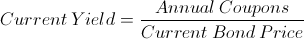# Current YieldCurrent yield is a bond's annual return based on its annual coupon payments and current price (as opposed to its original price or face). The formula for current yield is a bond's annual coupons divided by its current price.

## Use of the Current Yield Formula

The current yield formula is used to determine the yield on a bond based on its current price. The current yield formula can be used along with the bond yield formula, yield to maturity, yield to call, and other bond yield formulas to compare the returns of various bonds.

The current yield formula may also be used with risk ratings and calculations to compare various bonds. As a general rule in financial theory, one would expect a higher premium, or return, for a riskier investment. If two bonds are held constant in respect to their risk, a higher return would be preferable.

## Current Yield vs. Other Bond Formulas

There are various formulas that are used to compare the yields on bonds. Current yield, as its name implies, is the current or 'here and now' annual yield based solely on coupons. This is the difference between the bond yield and current yield. The bond yield looks at the original price of the bond or face value.

The current yield differs from the yield to maturity in that the yield to maturity looks at all future inflows, including a higher or lower face value than its current price, to determine the yield based on a present value equal to the current price of the bond. The formula for current yield only looks at the current price and one year coupons.

## Example of the Current Yield Formula

An example of the current yield formula would be a bond that was issued at \$1,000 that has an aggregate annual coupon of \$100. The bond yield on this particular bond would be 10%. Suppose that the same bond is currently selling for \$900 based on today's market rates. Recall that if the price of a bond goes down, the market rates or bond rate has gone up. For this example, the current yield formula would be shown aswhich would return a current yield of 11.11%.

New to Finance?